WON plate131 | World!OfNumbers[ May 3, 2002 ] Powers of Palindromes as products of integers and their reversals Carlos Rivera Carlos constructed some general formulas relating palindromes & reversible numbers in a most interesting manner : (1)n(4)n(4)n * (4)n(4)n(1)n = [ (2)n(5)n(2)n ]2 (144)n * (441)n = [ (252)n ]2 1(0)n4(0)n4 * 4(0)n4(0)n1 = [ 2(0)n5(0)n2 ]2 15(9)n84 * 48(9)n51 = [ 27(9)n72 ]2 And the puzzle is... to find some other general formulas ![ May 5, 2002 ]Jean Claude Rosa [email] sends three other formulasof which the first two resemble those of Carlos. 144(0)n144 * 441(0)n441 = [ 252(0)n252 ]2 1(0)n5(0)n8(0)n4 * 4(0)n8(0)n5(0)n1 = [ 2(0)n7(0)n7(0)n2 ]2 276(36)n48 * 84(63)n672 = [ 48(36)n384 ]2[ May 7-8-11, 2002 ]Jean Claude Rosa finds it a good fun puzzle and submits more of the same. 158(558)n4 * 4(855)n851 = [ 277(477)n2 ]2 144(0)n480(0)n4 * 4(0)n084(0)n441 = [ 24(0)n292(0)n42 ]2 (1)n0(4)n0(4)n * (4)n0(4)n0(1)n = [ (2)n0(5)n0(2)n ]2 1(45)n44 * 44(54)n1 = [ 25(45)n2 ]2 (1)n(5)n(8)n(4)n * (4)n(8)n(5)n(1)n = [ (2)n(77)n(2)n ]2 148(988)n84 * 48(889)n841 = [ 269(889)n62 ]2 14(558)n544 * 445(855)n41 = [ 254(774)n52 ]2 "et la suivante, très jolie et très simple" (1584)n * (4851)n = [ (2772)n ]2[ May 11-12-13, 2002 ] Despite being thrilled with all his previous discoveries Jean Claude Rosa took some distance from his equations only to notice that some generalisations could be made ! First he sampled the next three methods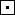Number repetition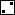Digit repetition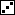Inserting zeros144 * 441 = 2522(144)n * (441)n = [(252)n]2(1)n(4)n(4)n * (4)n(4)n(1)n = [(2)n(5)n(2)n]21(0)n4(0)n4 * 4(0)n4(0)n1 = [2(0)n5(0)n2]21584 * 4851 = 27722(1584)n * (4851)n = [(2772)n]2(1)n(5)n(8)n(4)n * (4)n(8)n(5)n(1)n = [(2)n(7)n(7)n(2)n]21(0)n5(0)n8(0)n4 * 4(0)n8(0)n5(0)n1 = [2(0)n7(0)n7(0)n2]212544 * 44521 = 236322(12544)n * (44521)n = [(23632)n]2(1)n(2)n(5)n(4)n(4)n * (4)n(4)n(5)n(2)n(1)n = [(2)n(3)n(6)n(3)n(2)n]21(0)n2(0)n5(0)n4(0)n4 * 4(0)n4(0)n5(0)n2(0)n1 = [2(0)n3(0)n6(0)n3(0)n2]2 From that data he generalized these results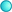If Q * R = P2   (R is the reverse of Q) then (Q)n * (R)n = [(P)n]2 is also true "The proof of this result is very easy", he adds.Let Q = abc...xyz ; R = zyx...cba ; P = klm...mlk then the equality Q * R = P2 leads to[(a)n(b)n(c)n...(x)n(y)n(z)n] * [(z)n(y)n(x)n...(c)n(b)n(a)n] = [(k)n(l)n(m)n...(m)n(l)n(k)n]2[a(0)nb(0)nc...x(0)ny(0)nz] * [z(0)ny(0)nx...c(0)nb(0)na] = [k(0)nl(0)nm...m(0)nl(0)nk]2 Here are the first ten basic Q numbers which can be applied generally to the three equation methods described above. (no other Q's up to 1,000,000 - the R's are not listed) 144 1584 12544 14544 14884 15984 27648 137984 159984 409739 Numbers like 10404, 114444 or 144144 are not included as basic either since these can be deduced from 144 by applying one or more of the three described methods. The three methods are applicable to any number (basic or not) but the order of application is important. Indeed we have method+ method= method+ methodmethod+ method= method+ methodbut method+ method<> method+ methodThe law of method composition is not commutative ! as the following example shows Let starting Q number be 1584 First method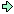11558844 and then methodgives101050508080404 First method1050804 and then methodgives11005500880044A000131Prime Curios!Prime PuzzleWikipedia 131Le nombre 131```

```

[TOP OF PAGE]

Patrick De Geest - Belgium- Short Bio - Some Pictures